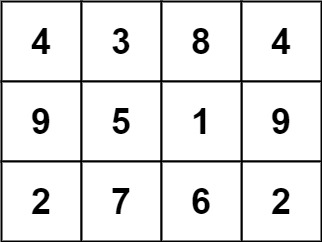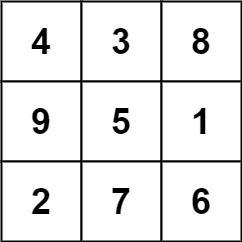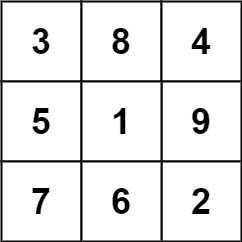## Algorithm

Problem Name: 840. Magic Squares In Grid

A `3 x 3` magic square is a `3 x 3` grid filled with distinct numbers from `1` to `9` such that each row, column, and both diagonals all have the same sum.

Given a `row x col` `grid` of integers, how many `3 x 3` "magic square" subgrids are there?  (Each subgrid is contiguous).

Example 1:```Input: grid = [[4,3,8,4],[9,5,1,9],[2,7,6,2]]
Output: 1
Explanation:
The following subgrid is a 3 x 3 magic square:while this one is not:In total, there is only one magic square inside the given grid.
```

Example 2:

```Input: grid = []
Output: 0
```

Constraints:

• `row == grid.length`
• `col == grid[i].length`
• `1 <= row, col <= 10`
• `0 <= grid[i][j] <= 15`

## Code Examples

### #1 Code Example with Python Programming

```Code - Python Programming```

``````
class Solution:
def numMagicSquaresInside(self, grid):
"""
:type grid: List[List[int]]
:rtype: int
"""
res = 0
for i in range(len(grid)-2):
for j in range(len(grid)-2):
if sum(grid[i][j: j + 3]) == sum(grid[i + 1][j : j +3]) == sum(grid[i + 2][j:j + 3]) == sum(grid[k][j] for k in range(i, i + 3)) == sum(grid[k][j + 1] for k in range(i, i + 3)) == sum(grid[k][j + 2] for k in range(i, i + 3)) == (grid[i][j] + grid[i + 1][j + 1] + grid[i + 2][j + 2]) == (grid[i+2][j]+ grid[i + 1][j + 1] + grid[i][j + 2]):
if set(grid[i][j: j + 3] + grid[i + 1][j: j +3] + grid[i + 2][j:j + 3]) == {1,2,3,4,5,6,7,8,9}: res += 1
return res

``````
Copy The Code &

Input

cmd
grid = [[4,3,8,4],[9,5,1,9],[2,7,6,2]]

Output

cmd
1

### #2 Code Example with C# Programming

```Code - C# Programming```

``````
namespace LeetCode
{
public class _0840_MagicSquaresInGrid
{
public int NumMagicSquaresInside(int[][] grid)
{
int rows = grid.Length, cols = grid.Length;
int ans = 0;
for (int r = 1; r < rows - 1; r++)
for (int c = 1; c < cols - 1; c++)
{
if (grid[r][c] != 5) continue;
if (IsMagic(new int[] { grid[r - 1][c - 1], grid[r - 1][c],     grid[r - 1][c + 1],
grid[r][c - 1],     grid[r][c],         grid[r][c + 1],
grid[r + 1][c - 1], grid[r + 1][c],     grid[r + 1][c + 1] }))
ans++;
}

return ans;
}

private bool IsMagic(int[] arr)
{
var count = new int;
foreach (int val in arr)
count[val]++;

for (int i = 1; i <= 9; i++)
if (count[i] != 1)
return false;

return (arr + arr + arr == 15 &&
arr + arr + arr == 15 &&
arr + arr + arr == 15 &&
arr + arr + arr == 15 &&
arr + arr + arr == 15 &&
arr + arr + arr == 15 &&
arr + arr + arr == 15 &&
arr + arr + arr == 15);
}
}
}
``````
Copy The Code &

Input

cmd
grid = [[4,3,8,4],[9,5,1,9],[2,7,6,2]]

Output

cmd
1